Скачать презентацию ELASTICITY OF DEMAND Degree of responsiveness of demand

9c8a873e4d1cc6e379419d4943222b2f.ppt

• Количество слайдов: 19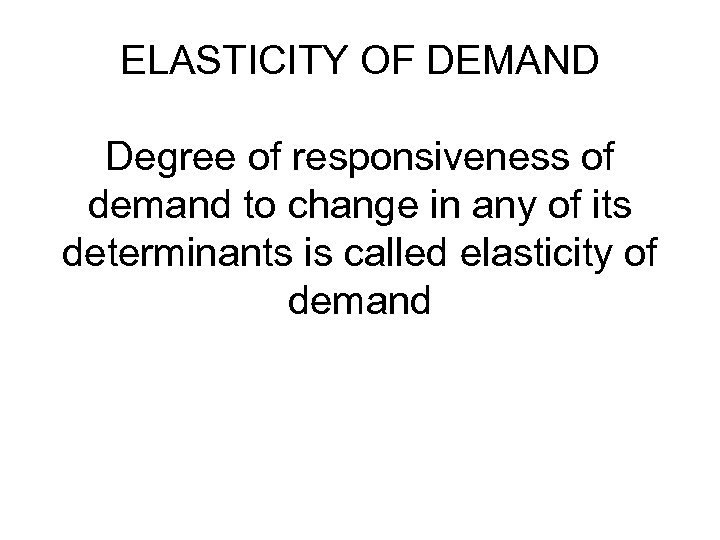ELASTICITY OF DEMAND Degree of responsiveness of demand to change in any of its determinants is called elasticity of demand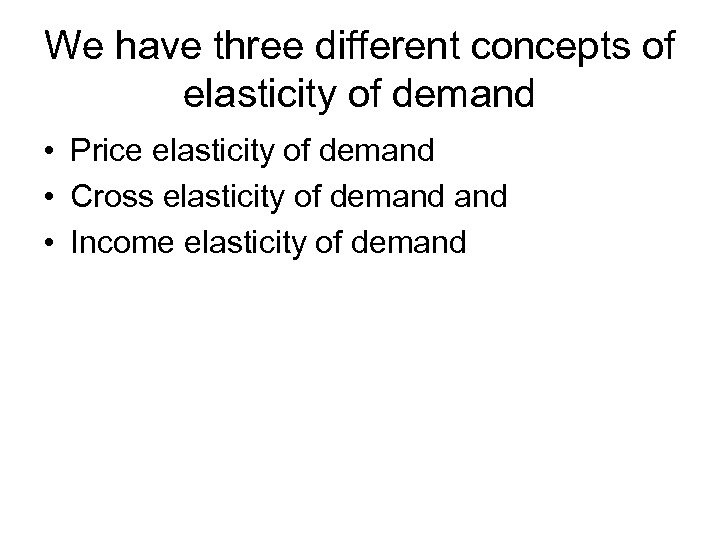We have three different concepts of elasticity of demand • Price elasticity of demand • Cross elasticity of demand • Income elasticity of demand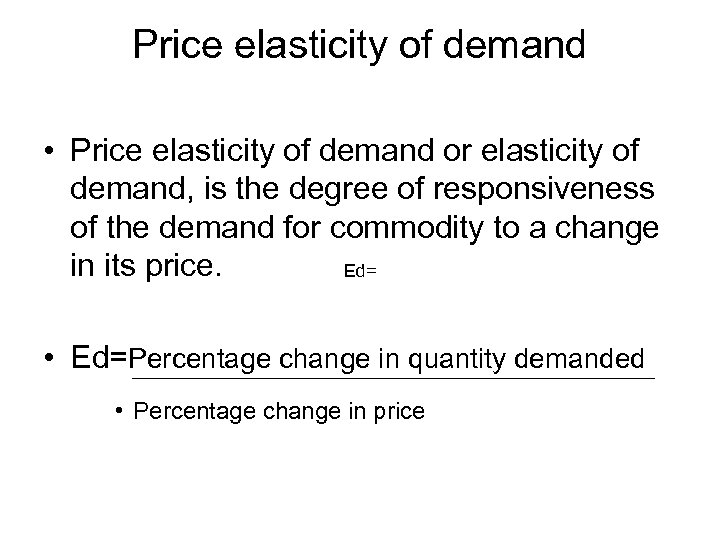Price elasticity of demand • Price elasticity of demand or elasticity of demand, is the degree of responsiveness of the demand for commodity to a change in its price. Ed= • Ed=Percentage change in quantity demanded • Percentage change in price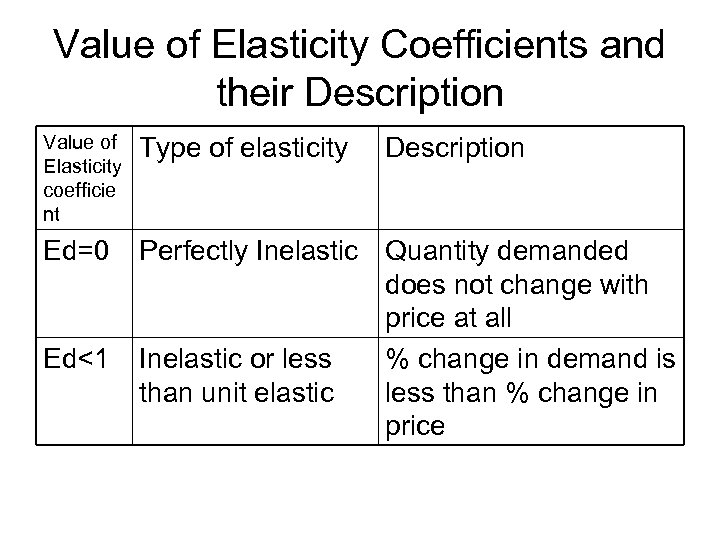Value of Elasticity Coefficients and their Description Value of Elasticity coefficie nt Type of elasticity Ed=0 Perfectly Inelastic Quantity demanded does not change with price at all Inelastic or less % change in demand is than unit elastic less than % change in price Ed<1 Description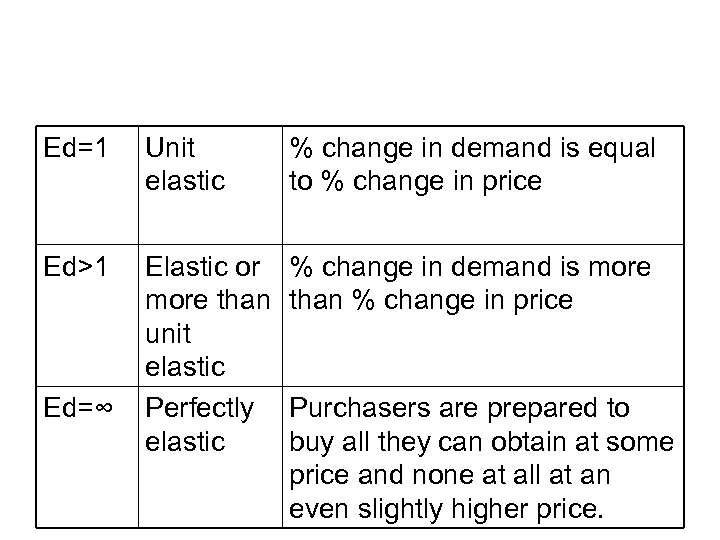Ed=1 Unit elastic % change in demand is equal to % change in price Ed>1 Elastic or more than unit elastic Perfectly elastic % change in demand is more than % change in price Ed=∞ Purchasers are prepared to buy all they can obtain at some price and none at all at an even slightly higher price.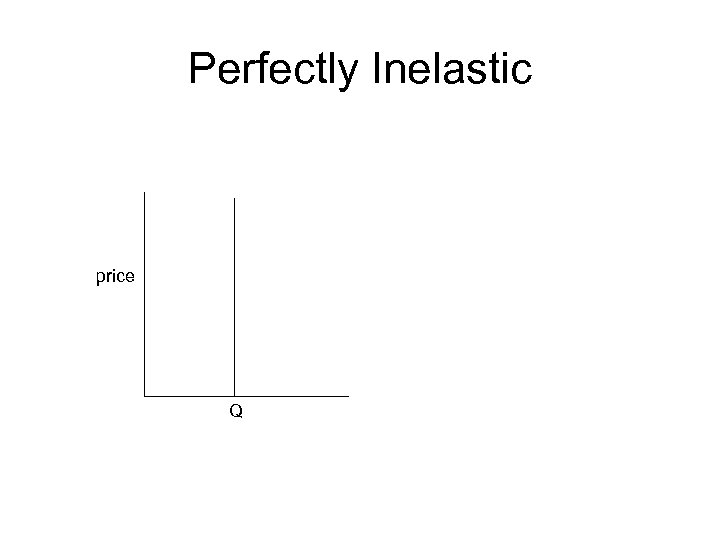Perfectly Inelastic price Q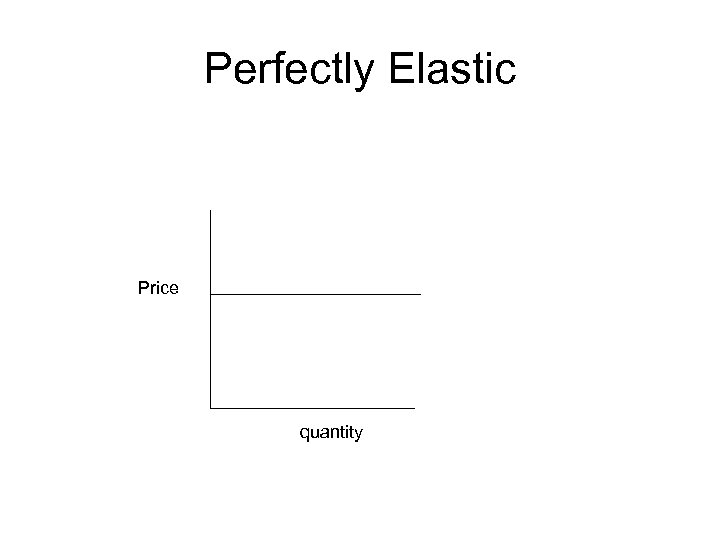Perfectly Elastic Price quantity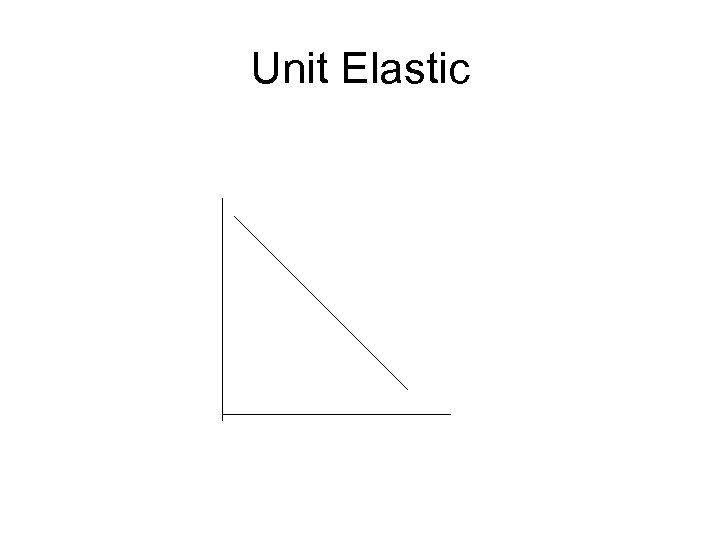Unit Elastic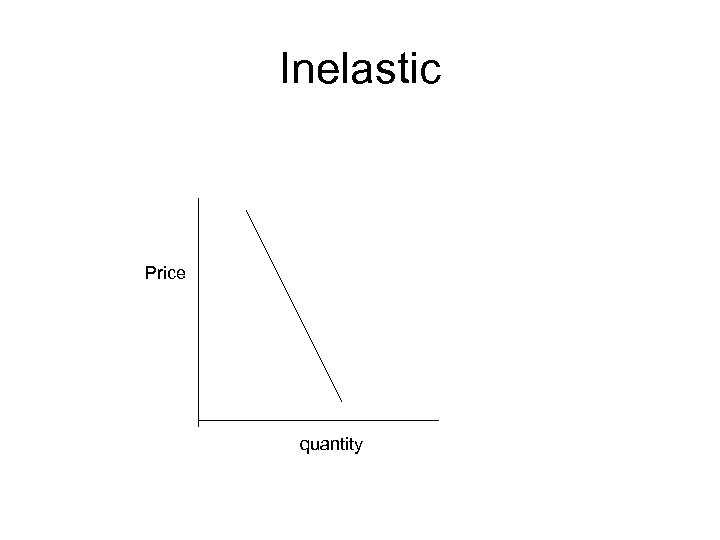Inelastic Price quantity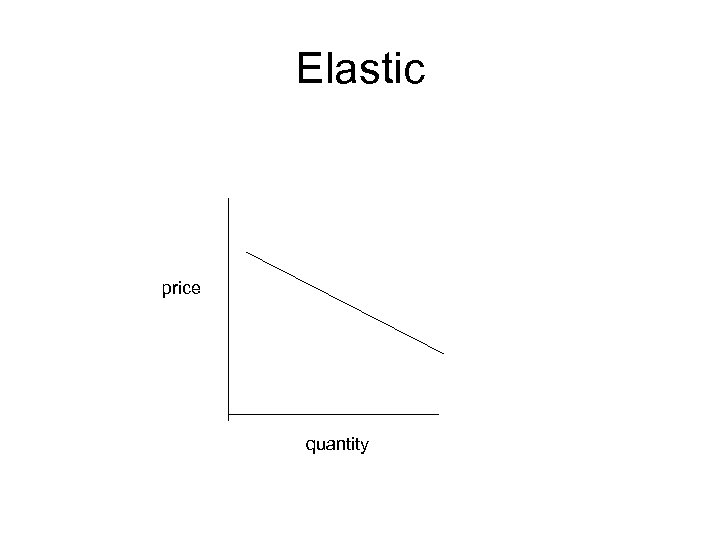Elastic price quantity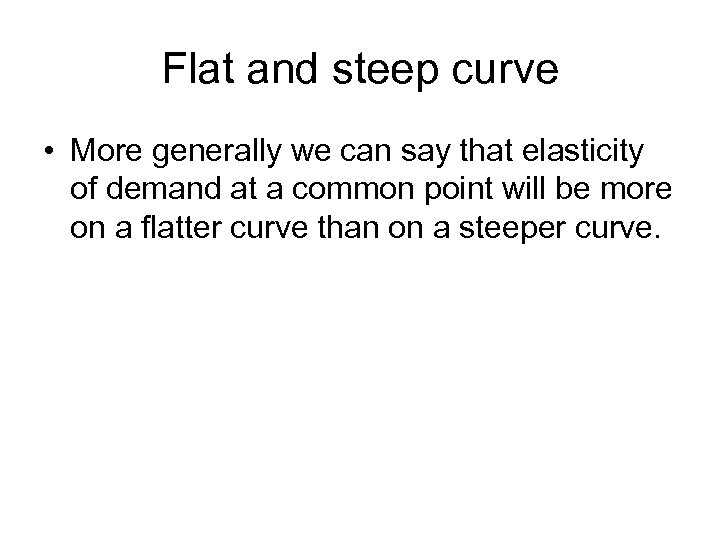Flat and steep curve • More generally we can say that elasticity of demand at a common point will be more on a flatter curve than on a steeper curve.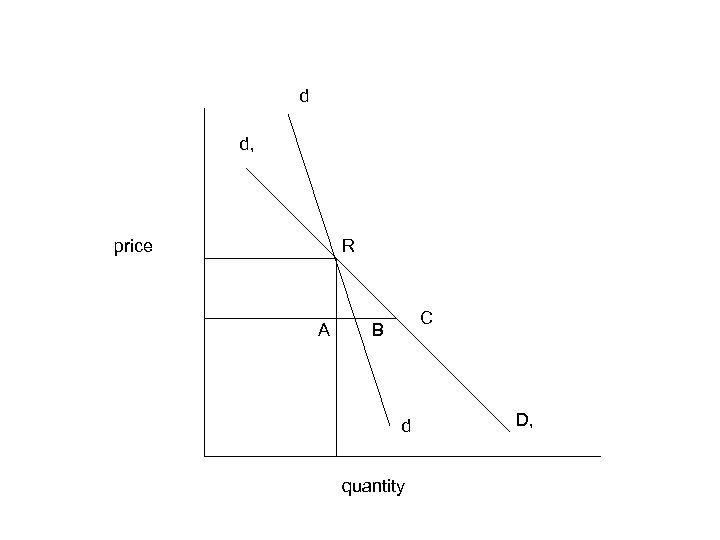d d, price R A C B d quantity D,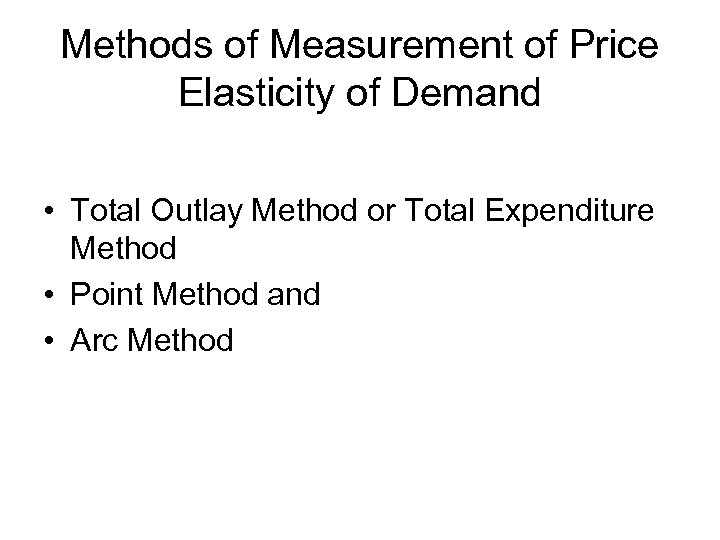Methods of Measurement of Price Elasticity of Demand • Total Outlay Method or Total Expenditure Method • Point Method and • Arc Method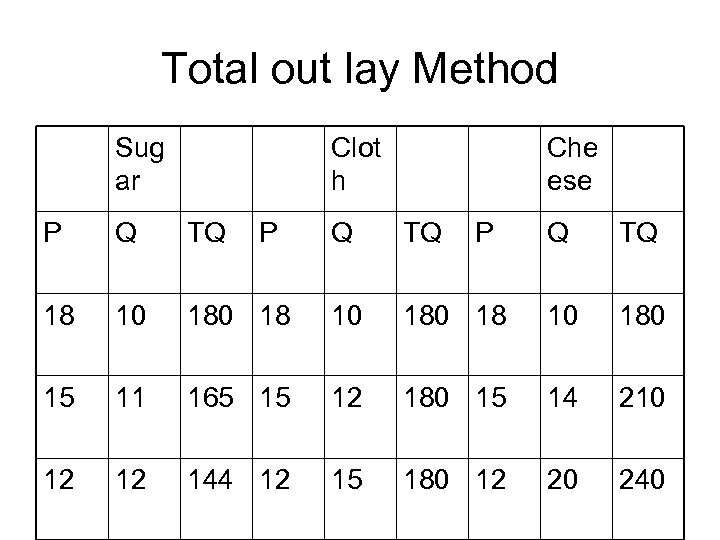Total out lay Method Sug ar Clot h P Q TQ 18 10 15 12 P Che ese Q TQ 180 18 10 11 165 15 12 144 12 P Q TQ 180 18 10 180 12 180 15 14 210 15 180 12 20 240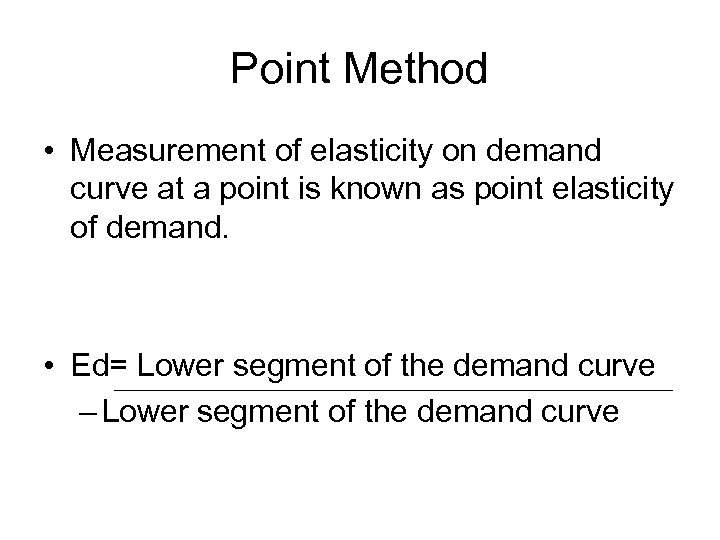Point Method • Measurement of elasticity on demand curve at a point is known as point elasticity of demand. • Ed= Lower segment of the demand curve – Lower segment of the demand curve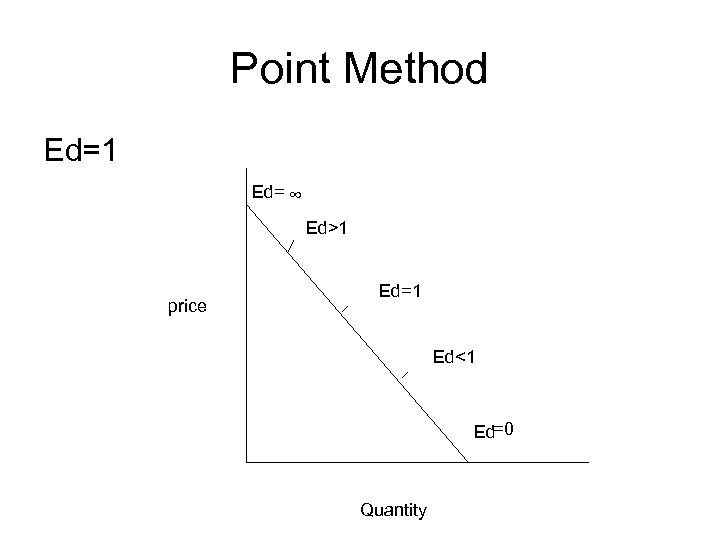Point Method Ed=1 Ed= ∞ Ed>1 price Ed=1 Ed<1 Ed=0 Quantity